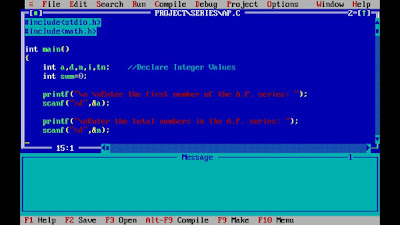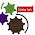## Wednesday, 23 December 2015

### Arithmetic Progression - Write A Program To Find SUM of AP C Programming Examples

We re starting C Programming Tutorial Series.We will try to satisfy our readers by giving some quality content in this series.

To Begin-with we have chosen to start with mathematical series.First series that always troubles students is Arithmetic progression.In this post you will find some basic idea and some useful concepts below.

Arithmetic Progression Step-wise Video Tutorial(See C- Codes Below ):

Integrated Environment : TURBO C/C++ - Getting Started
TURBO C/C++: Installer and Installation
C Programming Basic Questions
Variables-Pointer-Array-Files
Write A Program To Find SUM of Geometric Progression
Write A Program To Find SUM of Infinite Harmonic Progression

Arithmetic Progression / Sequence  Mathematically Explained:

Arithmetic progression (AP) is a sequence of numbers in which each term after the first is obtained by adding a constant, d to the preceding term. The constant d is called common difference.

An arithmetic progression is given by a, (a + d), (a + 2d), (a + 3d), ...
where a = the first term , d = the common difference

Sum of first N Terms of AP:

Nth term of an arithmetic progression Tn = a + (n – 1)d
(where Tn = nth term, a= the first term , d= common difference)

Number of terms of an arithmetic progression
n=(l−a)d+1
(where n = number of terms, a= the first term , l = last term, d= common difference)
To solve most of the problems related to A.P., the terms can be conveniently taken as

1. Third terms : (a – d), a, (a +d)
2. Fourth terms : (a – 3d), (a – d), (a + d), (a +3d)
3. Fifth terms : (a – 2d), (a – d), a, (a + d), (a +2d)
4. Nth Term=Tn = Sn - Sn-1
5. If each term of an A.P. is increased, decreased , multiplied or divided by the same non-zero constant, the resulting sequence also will be in A.P.
6. In an A.P., sum of terms equidistant from beginning and end will be constantWrite A Program To Find SUM of Arithmetic Progression / Sequence

C Codes are written and tested in TuboC/C++ IDE as you can see in Video:

#include<stdio.h>
#include<math.h>

int main()
{
int a,d,n,i,tn;    //Declare Integer Values
int sum=0;

printf("\n \nEnter the first number of the A.P. series: ");
scanf("%d",&a);

printf("\nEnter the total numbers in the A.P. series: ");
scanf("%d",&n);

printf("\nEnter the common difference of A.P. series: ");
scanf("%d",&d);

sum = ( n * ( 2 * a + ( n -1 ) * d ) )/ 2; //Formula for Calculating Sum Of AP
tn = a + (n-1) * d;  // nth Term of the AP

printf("\n Sum of the series A.P.: ");

for(i=a;i<=tn; i= i + d ) //Calculation of Sum of the series
{
if (i != tn)
printf("%d + ",i);
else
printf("%d = %d ",i,sum);
}
delay(5000);    //To Display Result on the Screen
return 0;
}

1.Even though we are lagging in, our friends might be happy with the post

2.3.4.